# Kissing the curve – the gruesome details

By
Rachel Thomas

So, you want to more? Here are all the gruesome details for calculating the tangent, normal, curvature and osculating circle of a curve!

Think of your favourite smooth curve in a plane that can be described by a function,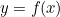. Then at any point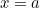, the slope of the tangent line is just the value of the derivative of the function at that point,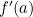. With this information, and the fact that the tangent must pass through the point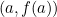, we can work out that the equation for the tangent is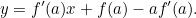The normal to the curve is perpendicular to the tangent, and so the product of their respective slopes must be -1. So the slope of the normal must be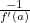. Again, with this information and the fact that the normal must also pass through the point, we can work out that the equation for the normal to the curve at this point is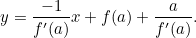The curvature of the curve is the rate at which the tangent changes direction as you move along the curve at constant speed. This is a little tricky to work out (you can see all the details at Wolfram MathWorld) but the curvature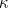of a curve at a pointis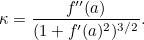From the curvature,we can calculate the radius of curvature,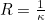. This is the radius of the osculating circle of the curve at the point. Now we know the radius of the osculating circle, we just need to calculate its centre. The centre lies on the normal line, and is a distance of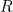from the point. If you use these two fact (and about 20 sheets of paper if you keep making silly mistakes like us!) then you can finally calculate the centre of the osculating circle to be the point: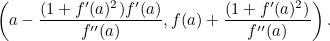And therefore, the equation for the osculating circle of the curve at the pointis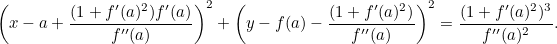Hooray!

Go back to the article or play with the worksheet below.

You can use this geogebra worksheet to see the tangent, normal and osculating circle of any smooth curve you choose - just change the equation f(x) in the left-hand panel.

Hi, thanks for this page and the accompanying article! I think in the tangent equation it should be -af'(a) not -f'(a)?

### Thanks for pointing that out,

Thanks for pointing that out, we have corrected the error.